# RMS VI

## LabVIEW 2018 Help

Edition Date: March 2018
Part Number: 371361R-01
View Product InfoLabVIEW 2016 HelpLabVIEW 2017 HelpLabVIEW 2018 HelpLabVIEW 2019 HelpLabVIEW 2020 Help

Owning Palette: Probability & Statistics VIs

Requires: Full Development System

Computes the root mean square (rms) of the input sequence X.

Use the pull-down menu to select an instance of this VI.

 Select an instance RMS (DBL)RMS (CDB)

## RMS (DBL)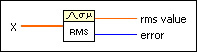X is the real input sequence. If X is empty, rms value is NaN.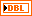rms value is the root mean square computed from the input sequence X.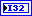error returns any error or warning from the VI. You can wire error to the Error Cluster From Error Code VI to convert the error code or warning into an error cluster.

## RMS (CDB)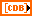X is the complex input sequence. If X is empty, rms value is NaN.rms value is the root mean square computed from the magnitudes of the input sequence X.error returns any error or warning from the VI. You can wire error to the Error Cluster From Error Code VI to convert the error code or warning into an error cluster.

## RMS Details

The VI calculates rms value using the following equation: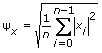where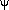x is rms value and n is the number of elements in X.

## Example

Refer to the Statistics Solver VI in the labview\examples\Mathematics\Probability and Statistics directory for an example of using the RMS VI.